# Java循环队列的介绍（代码示例）发布日期：2019-04-27  来源：IdW2RN  作者：xinb2b.cn  浏览次数：93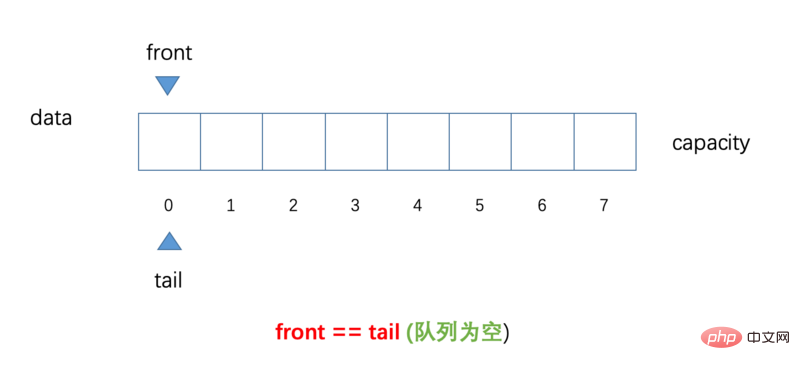`front`：表示队列队首，始终指向队列中的第一个元素（当队列空时，front指向索引为0的位置）

`tail`：表示队列队尾，始终指向队列中的最后一个元素的下一个位置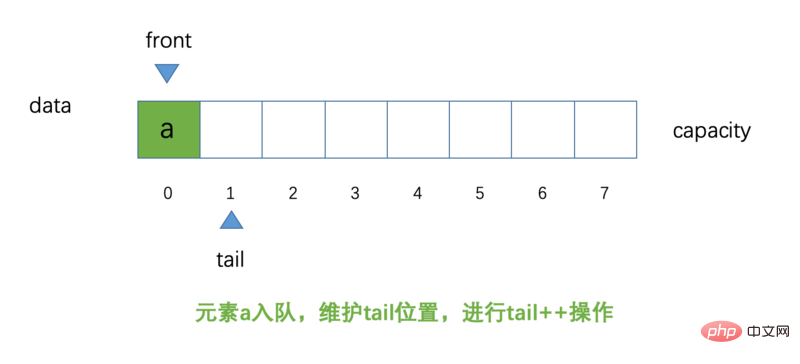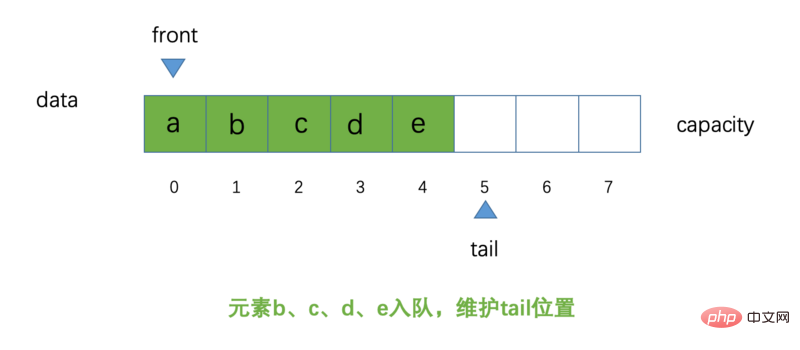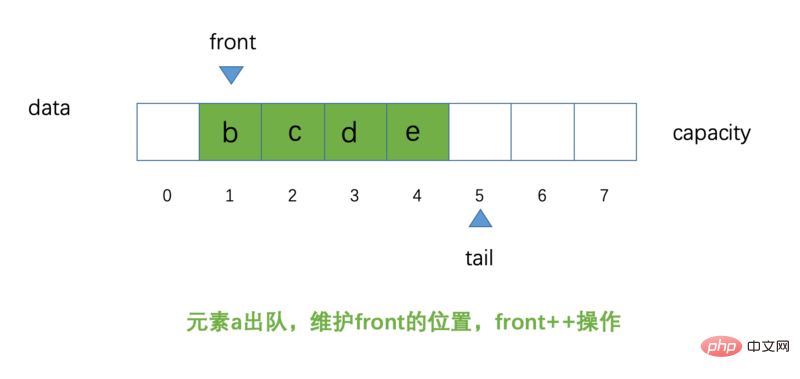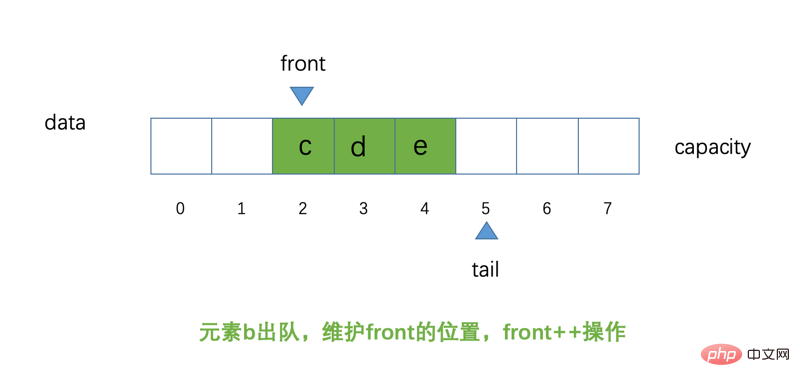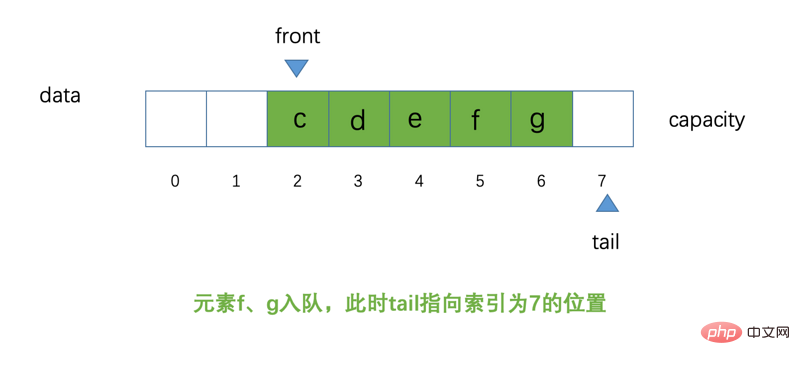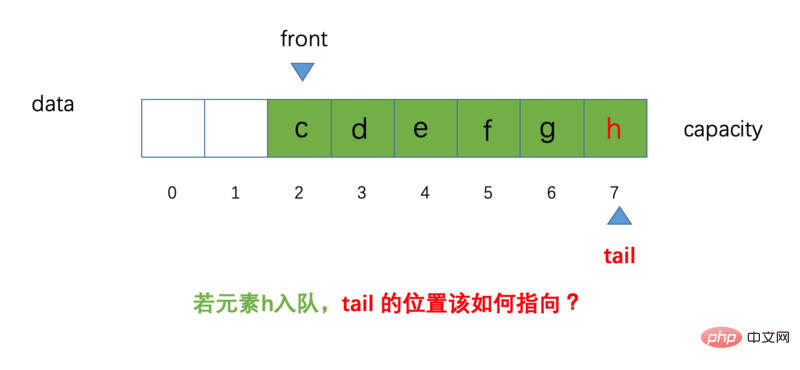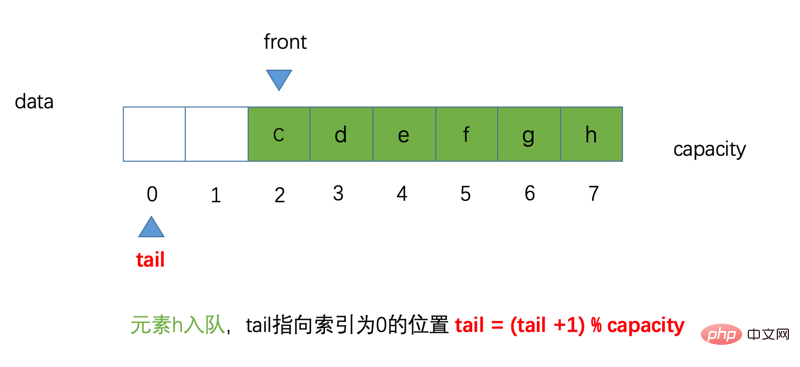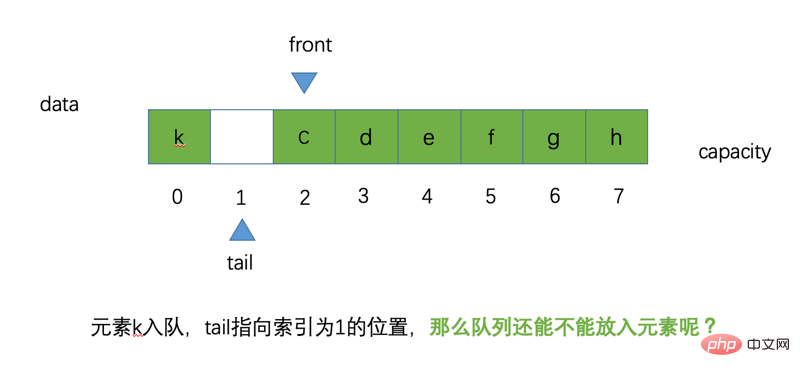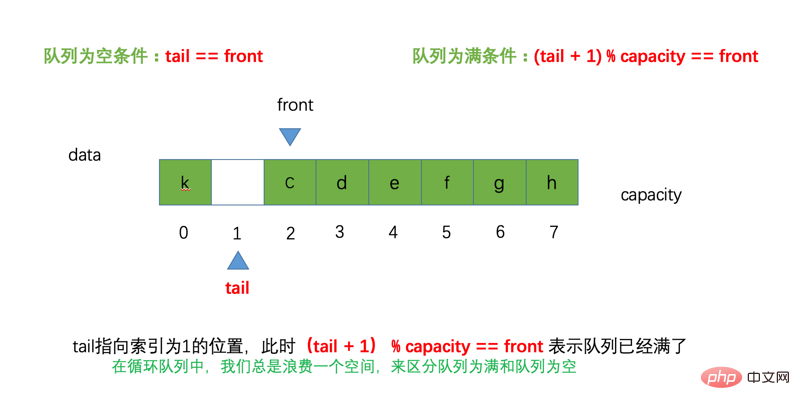```public interface Queue<E> {

void enqueue(E e);

E dequeue();

E getFront();

int getSize();

boolean isEmpty();
}```

```public class LoopQueue<E> implements Queue<E> {

private E[] data;

private int front;

private int tail;

private int size;

public LoopQueue(int capacity) {
data = (E[]) new Object[capacity + 1];
}

public LoopQueue() {
this(10);
}

@Override
public void enqueue(E e) {
// 检查队列为满
if ((tail + 1) % data.length == front) {
// 队列扩容
resize(getCapacity() * 2);
}
data[tail] = e;
tail = (tail + 1) % data.length;
size++;
}

@Override
public E dequeue() {
if (isEmpty()) {
throw new IllegalArgumentException("队列为空");
}
// 出队元素
E element = data[front];
// 元素出队后，将空间置为null
data[front] = null;
// 维护front的索引位置(循环队列)
front = (front + 1) % data.length;
// 维护size大小
size--;

// 元素出队后，可以指定条件，进行缩容
if (size == getCapacity() / 2 && getCapacity() / 2 != 0) {
resize(getCapacity() / 2);
}
return element;
}

@Override
public E getFront() {
if (isEmpty()) {
throw new IllegalArgumentException("队列为空");
}
return data[front];
}

@Override
public int getSize() {
return size;
}

@Override
public boolean isEmpty() {
return front == tail;
}

// 队列快满时，队列扩容；元素出队操作，指定条件可以进行缩容
private void resize(int newCapacity) {
// 这里的加1还是因为循环队列我们在实际使用的过程中要浪费一个空间
E[] newData = (E[]) new Object[newCapacity + 1];
for (int i = 0; i < size; i++) {
// 注意这里的写法：因为在数组中，front 可能不是在索引为0的位置，相对于i有一个偏移量
newData[i] = data[(i + front) % data.length];
}
// 将新的数组引用赋予原数组的指向
data = newData;
// 充值front的位置（front总是指向队列中第一个元素）
front = 0;
// size 的大小不变，因为在这过程中，没有元素入队和出队
tail = size;
}

private int getCapacity() {
// 注意：在初始化队列的时候，我们有意识的为队列加了一个空间，那么它的实际容量自然要减1
return data.length - 1;
}

@Override
public String toString() {
return "LoopQueue{" +
"【队首】data=" + Arrays.toString(data) + "【队尾】" +
", front=" + front +
", tail=" + tail +
", size=" + size +
", capacity=" + getCapacity() +
'}';
}
}```

```public class LoopQueueTest {
@Test
public void testLoopQueue() {
LoopQueue<Integer> loopQueue = new LoopQueue<>();
for (int i = 0; i < 10; i++) {
loopQueue.enqueue(i);
}
// 初始化队列数据
System.out.println("原始队列: " + loopQueue);
// 元素0出队
loopQueue.dequeue();
System.out.println("元素0出队: " + loopQueue);
loopQueue.dequeue();
System.out.println("元素1出队: " + loopQueue);
loopQueue.dequeue();
System.out.println("元素2出队: " + loopQueue);
loopQueue.dequeue();
System.out.println("元素3出队: " + loopQueue);
loopQueue.dequeue();
System.out.println("元素4出队,发生缩容: " + loopQueue);
// 队首元素
System.out.println("队首元素：" + loopQueue.getFront());
}
}```
##### 测试结果：
```原始队列: LoopQueue{【队首】data=[0, 1, 2, 3, 4, 5, 6, 7, 8, 9, null]【队尾】, front=0, tail=10, size=10, capacity=10}

[ 编程语言搜索 ]  [ 加入收藏 ]  [ 告诉好友 ]  [ 打印本文 ]  [ 违规举报 ]  [ 关闭窗口 ]